# Scalable Data Science in Python and R on Apache Spark

8 Jul 2017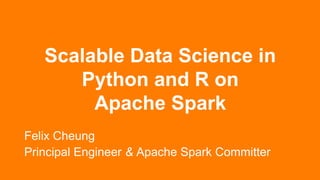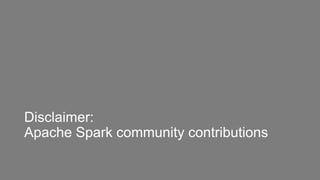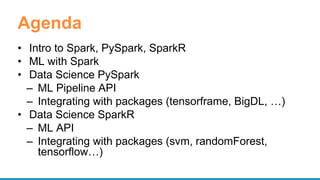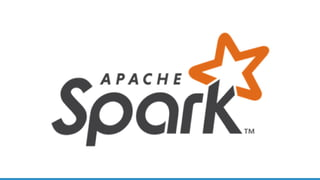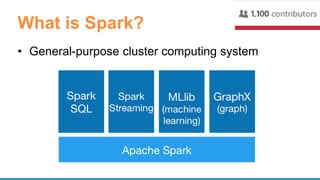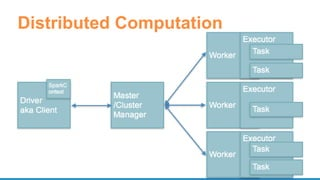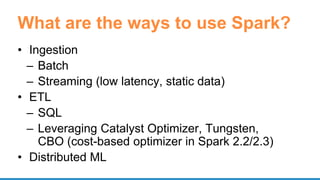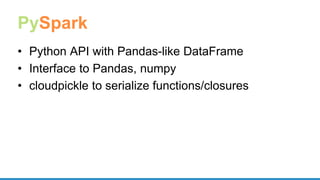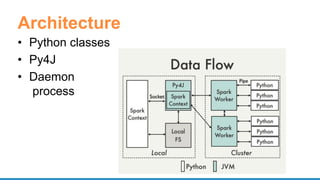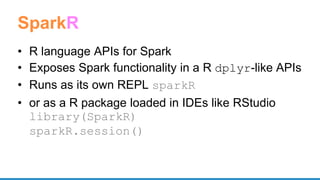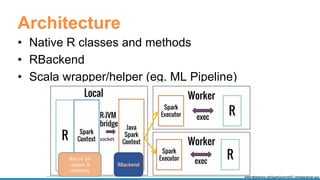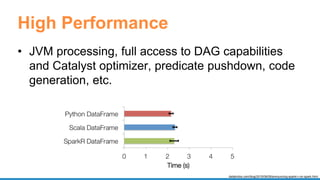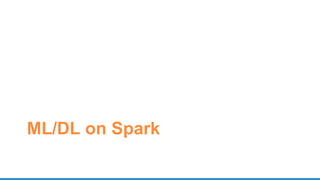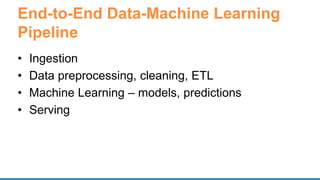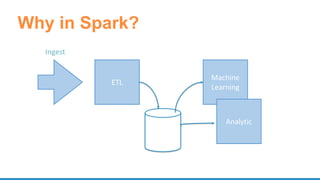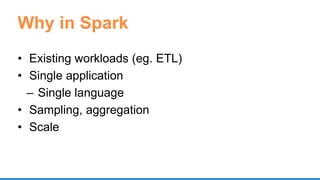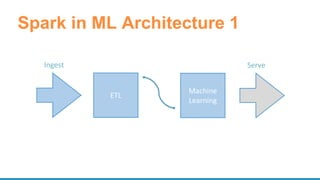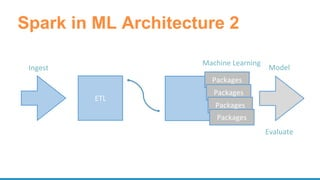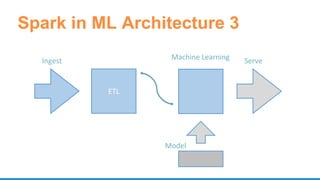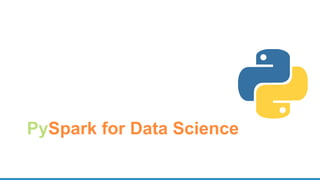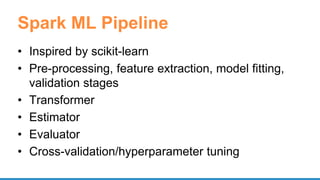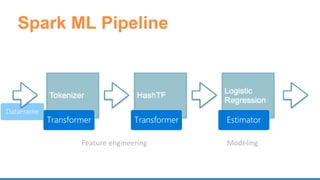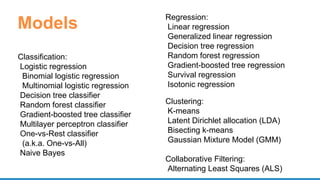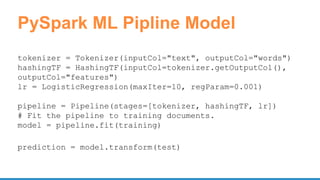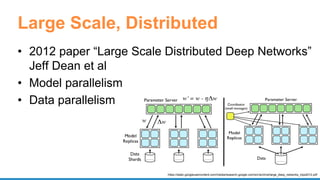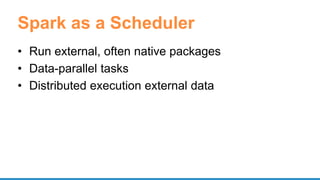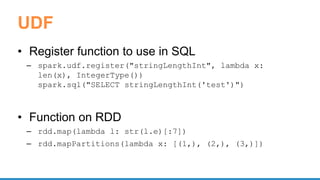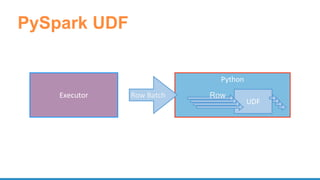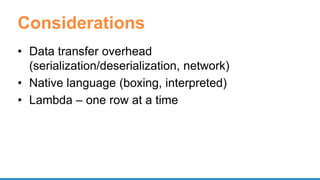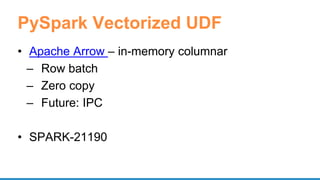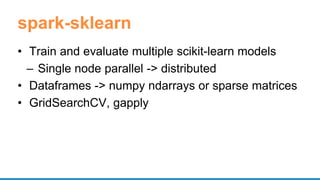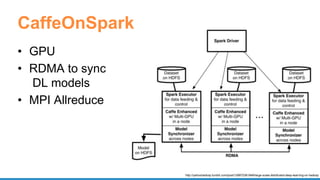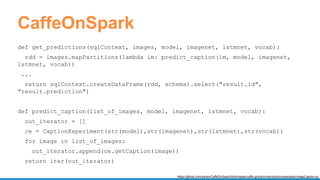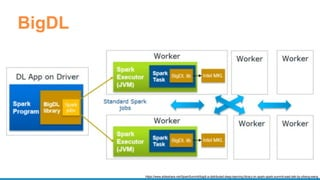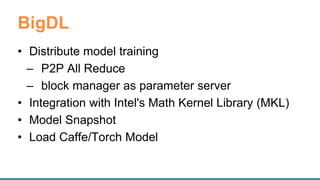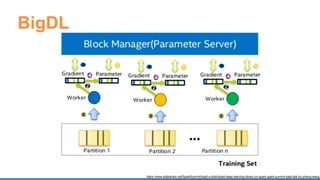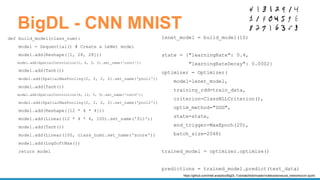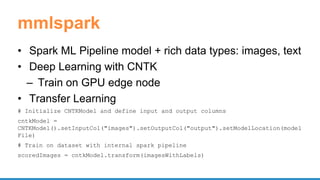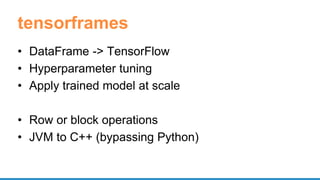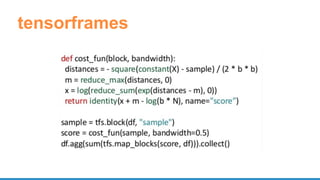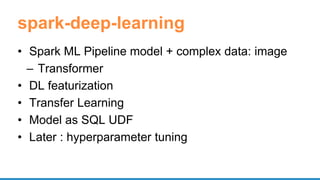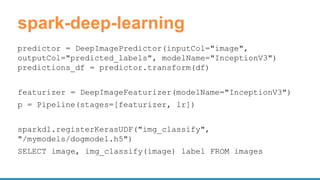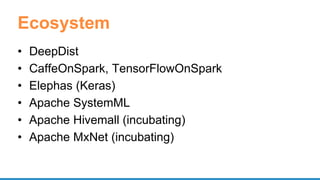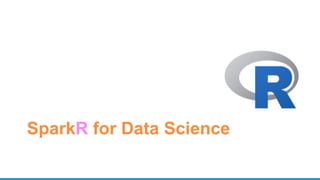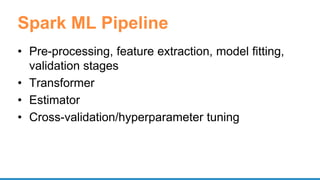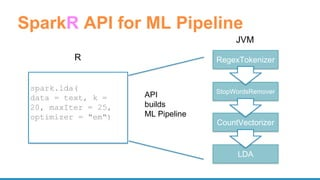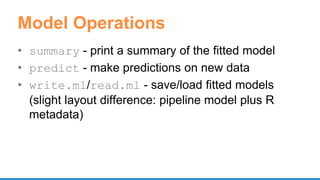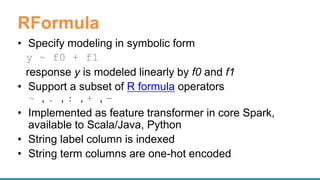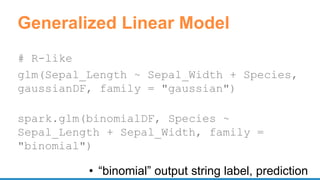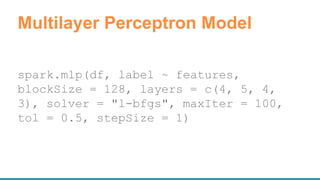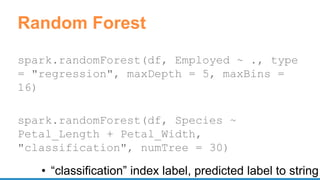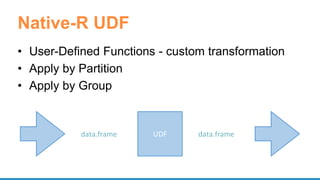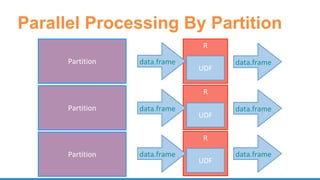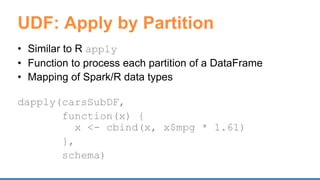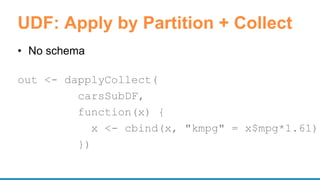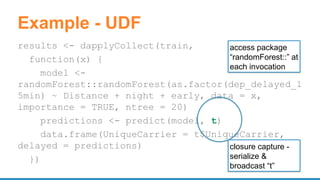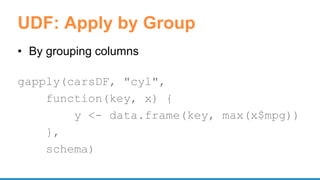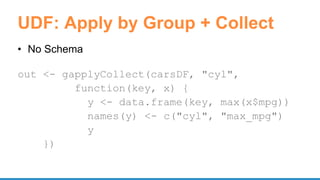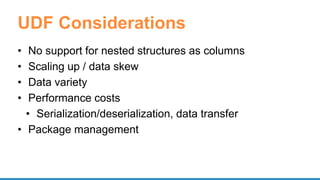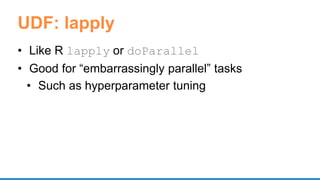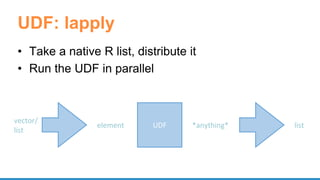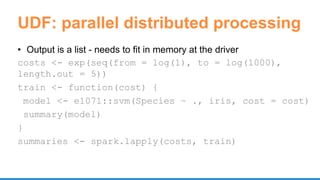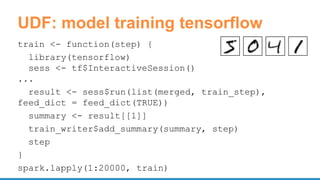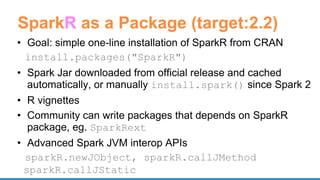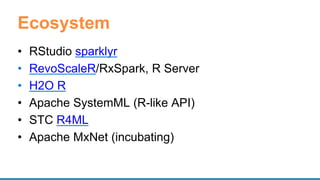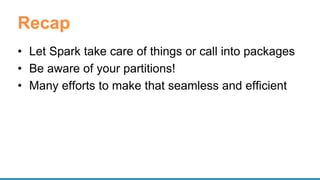1 sur 68

### Scalable Data Science in Python and R on Apache Spark

• 1. Scalable Data Science in Python and R on Apache Spark Felix Cheung Principal Engineer & Apache Spark Committer
• 3. Agenda • Intro to Spark, PySpark, SparkR • ML with Spark • Data Science PySpark – ML Pipeline API – Integrating with packages (tensorframe, BigDL, …) • Data Science SparkR – ML API – Integrating with packages (svm, randomForest, tensorflow…)
• 5. What is Spark? • General-purpose cluster computing system
• 7. What are the ways to use Spark? • Ingestion – Batch – Streaming (low latency, static data) • ETL – SQL – Leveraging Catalyst Optimizer, Tungsten, CBO (cost-based optimizer in Spark 2.2/2.3) • Distributed ML
• 9. PySpark • Python API with Pandas-like DataFrame • Interface to Pandas, numpy • cloudpickle to serialize functions/closures
• 10. Architecture • Python classes • Py4J • Daemon process
• 11. SparkR • R language APIs for Spark • Exposes Spark functionality in a R dplyr-like APIs • Runs as its own REPL sparkR • or as a R package loaded in IDEs like RStudio library(SparkR) sparkR.session()
• 12. Architecture • Native R classes and methods • RBackend • Scala wrapper/helper (eg. ML Pipeline) www.slideshare.net/SparkSummit/07-venkataraman-sun
• 13. High Performance • JVM processing, full access to DAG capabilities and Catalyst optimizer, predicate pushdown, code generation, etc. databricks.com/blog/2015/06/09/announcing-sparkr-r-on-spark.html
• 15. End-to-End Data-Machine Learning Pipeline • Ingestion • Data preprocessing, cleaning, ETL • Machine Learning – models, predictions • Serving
• 17. Why in Spark • Existing workloads (eg. ETL) • Single application – Single language • Sampling, aggregation • Scale
• 18. Spark in ML Architecture 1 ETL Ingest Machine Learning Serve
• 19. Spark in ML Architecture 2 ETL Ingest Model Packages Packages Packages Packages Machine Learning Evaluate
• 20. Spark in ML Architecture 3 ETL Ingest Model Machine Learning Serve
• 21. PySpark for Data Science
• 22. Spark ML Pipeline • Inspired by scikit-learn • Pre-processing, feature extraction, model fitting, validation stages • Transformer • Estimator • Evaluator • Cross-validation/hyperparameter tuning
• 23. DataFrame Spark ML Pipeline Transformer EstimatorTransformer Feature engineering Modeling
• 24. Classification: Logistic regression Binomial logistic regression Multinomial logistic regression Decision tree classifier Random forest classifier Gradient-boosted tree classifier Multilayer perceptron classifier One-vs-Rest classifier (a.k.a. One-vs-All) Naive Bayes Models Clustering: K-means Latent Dirichlet allocation (LDA) Bisecting k-means Gaussian Mixture Model (GMM) Collaborative Filtering: Alternating Least Squares (ALS) Regression: Linear regression Generalized linear regression Decision tree regression Random forest regression Gradient-boosted tree regression Survival regression Isotonic regression
• 25. PySpark ML Pipline Model tokenizer = Tokenizer(inputCol="text", outputCol="words") hashingTF = HashingTF(inputCol=tokenizer.getOutputCol(), outputCol="features") lr = LogisticRegression(maxIter=10, regParam=0.001) pipeline = Pipeline(stages=[tokenizer, hashingTF, lr]) # Fit the pipeline to training documents. model = pipeline.fit(training) prediction = model.transform(test)
• 26. Large Scale, Distributed • 2012 paper “Large Scale Distributed Deep Networks” Jeff Dean et al • Model parallelism • Data parallelism https://static.googleusercontent.com/media/research.google.com/en//archive/large_deep_networks_nips2012.pdf
• 27. Spark as a Scheduler • Run external, often native packages • Data-parallel tasks • Distributed execution external data
• 28. UDF • Register function to use in SQL – spark.udf.register("stringLengthInt", lambda x: len(x), IntegerType()) spark.sql("SELECT stringLengthInt('test')") • Function on RDD – rdd.map(lambda l: str(l.e)[:7]) – rdd.mapPartitions(lambda x: [(1,), (2,), (3,)])
• 30. Considerations • Data transfer overhead (serialization/deserialization, network) • Native language (boxing, interpreted) • Lambda – one row at a time
• 31. PySpark Vectorized UDF • Apache Arrow – in-memory columnar – Row batch – Zero copy – Future: IPC • SPARK-21190
• 32. spark-sklearn • Train and evaluate multiple scikit-learn models – Single node parallel -> distributed • Dataframes -> numpy ndarrays or sparse matrices • GridSearchCV, gapply
• 33. CaffeOnSpark • GPU • RDMA to sync DL models • MPI Allreduce http://yahoohadoop.tumblr.com/post/129872361846/large-scale-distributed-deep-learning-on-hadoop
• 34. CaffeOnSpark def get_predictions(sqlContext, images, model, imagenet, lstmnet, vocab): rdd = images.mapPartitions(lambda im: predict_caption(im, model, imagenet, lstmnet, vocab)) ... return sqlContext.createDataFrame(rdd, schema).select("result.id", "result.prediction") def predict_caption(list_of_images, model, imagenet, lstmnet, vocab): out_iterator = [] ce = CaptionExperiment(str(model),str(imagenet),str(lstmnet),str(vocab)) for image in list_of_images: out_iterator.append(ce.getCaption(image)) return iter(out_iterator) https://github.com/yahoo/CaffeOnSpark/blob/master/caffe-grid/src/main/python/examples/ImageCaption.py
• 36. BigDL • Distribute model training – P2P All Reduce – block manager as parameter server • Integration with Intel's Math Kernel Library (MKL) • Model Snapshot • Load Caffe/Torch Model
• 39. mmlspark • Spark ML Pipeline model + rich data types: images, text • Deep Learning with CNTK – Train on GPU edge node • Transfer Learning # Initialize CNTKModel and define input and output columns cntkModel = CNTKModel().setInputCol("images").setOutputCol("output").setModelLocation(model File) # Train on dataset with internal spark pipeline scoredImages = cntkModel.transform(imagesWithLabels)
• 40. tensorframes • DataFrame -> TensorFlow • Hyperparameter tuning • Apply trained model at scale • Row or block operations • JVM to C++ (bypassing Python)
• 42. spark-deep-learning • Spark ML Pipeline model + complex data: image – Transformer • DL featurization • Transfer Learning • Model as SQL UDF • Later : hyperparameter tuning
• 43. spark-deep-learning predictor = DeepImagePredictor(inputCol="image", outputCol="predicted_labels", modelName="InceptionV3") predictions_df = predictor.transform(df) featurizer = DeepImageFeaturizer(modelName="InceptionV3") p = Pipeline(stages=[featurizer, lr]) sparkdl.registerKerasUDF("img_classify", "/mymodels/dogmodel.h5") SELECT image, img_classify(image) label FROM images
• 44. Ecosystem • DeepDist • CaffeOnSpark, TensorFlowOnSpark • Elephas (Keras) • Apache SystemML • Apache Hivemall (incubating) • Apache MxNet (incubating)
• 45. SparkR for Data Science
• 46. Spark ML Pipeline • Pre-processing, feature extraction, model fitting, validation stages • Transformer • Estimator • Cross-validation/hyperparameter tuning
• 47. SparkR API for ML Pipeline spark.lda( data = text, k = 20, maxIter = 25, optimizer = "em") RegexTokenizer StopWordsRemover CountVectorizer R JVM LDA API builds ML Pipeline
• 48. Model Operations • summary - print a summary of the fitted model • predict - make predictions on new data • write.ml/read.ml - save/load fitted models (slight layout difference: pipeline model plus R metadata)
• 49. RFormula • Specify modeling in symbolic form y ~ f0 + f1 response y is modeled linearly by f0 and f1 • Support a subset of R formula operators ~ , . , : , + , - • Implemented as feature transformer in core Spark, available to Scala/Java, Python • String label column is indexed • String term columns are one-hot encoded
• 50. Generalized Linear Model # R-like glm(Sepal_Length ~ Sepal_Width + Species, gaussianDF, family = "gaussian") spark.glm(binomialDF, Species ~ Sepal_Length + Sepal_Width, family = "binomial") • “binomial” output string label, prediction
• 51. Multilayer Perceptron Model spark.mlp(df, label ~ features, blockSize = 128, layers = c(4, 5, 4, 3), solver = "l-bfgs", maxIter = 100, tol = 0.5, stepSize = 1)
• 52. Random Forest spark.randomForest(df, Employed ~ ., type = "regression", maxDepth = 5, maxBins = 16) spark.randomForest(df, Species ~ Petal_Length + Petal_Width, "classification", numTree = 30) • “classification” index label, predicted label to string
• 53. Native-R UDF • User-Defined Functions - custom transformation • Apply by Partition • Apply by Group UDFdata.frame data.frame
• 54. Parallel Processing By Partition R R R Partition Partition Partition UDF UDF UDF data.frame data.frame data.frame data.frame data.frame data.frame
• 55. UDF: Apply by Partition • Similar to R apply • Function to process each partition of a DataFrame • Mapping of Spark/R data types dapply(carsSubDF, function(x) { x <- cbind(x, x\$mpg * 1.61) }, schema)
• 56. UDF: Apply by Partition + Collect • No schema out <- dapplyCollect( carsSubDF, function(x) { x <- cbind(x, "kmpg" = x\$mpg*1.61) })
• 57. Example - UDF results <- dapplyCollect(train, function(x) { model <- randomForest::randomForest(as.factor(dep_delayed_1 5min) ~ Distance + night + early, data = x, importance = TRUE, ntree = 20) predictions <- predict(model, t) data.frame(UniqueCarrier = t\$UniqueCarrier, delayed = predictions) }) closure capture - serialize & broadcast “t” access package “randomForest::” at each invocation
• 58. UDF: Apply by Group • By grouping columns gapply(carsDF, "cyl", function(key, x) { y <- data.frame(key, max(x\$mpg)) }, schema)
• 59. UDF: Apply by Group + Collect • No Schema out <- gapplyCollect(carsDF, "cyl", function(key, x) { y <- data.frame(key, max(x\$mpg)) names(y) <- c("cyl", "max_mpg") y })
• 60. UDF Considerations • No support for nested structures as columns • Scaling up / data skew • Data variety • Performance costs • Serialization/deserialization, data transfer • Package management
• 61. UDF: lapply • Like R lapply or doParallel • Good for “embarrassingly parallel” tasks • Such as hyperparameter tuning
• 62. UDF: lapply • Take a native R list, distribute it • Run the UDF in parallel UDFelement *anything* vector/ list list
• 63. UDF: parallel distributed processing • Output is a list - needs to fit in memory at the driver costs <- exp(seq(from = log(1), to = log(1000), length.out = 5)) train <- function(cost) { model <- e1071::svm(Species ~ ., iris, cost = cost) summary(model) } summaries <- spark.lapply(costs, train)
• 64. UDF: model training tensorflow train <- function(step) { library(tensorflow) sess <- tf\$InteractiveSession() ... result <- sess\$run(list(merged, train_step), feed_dict = feed_dict(TRUE)) summary <- result[] train_writer\$add_summary(summary, step) step } spark.lapply(1:20000, train)
• 65. SparkR as a Package (target:2.2) • Goal: simple one-line installation of SparkR from CRAN install.packages("SparkR") • Spark Jar downloaded from official release and cached automatically, or manually install.spark() since Spark 2 • R vignettes • Community can write packages that depends on SparkR package, eg. SparkRext • Advanced Spark JVM interop APIs sparkR.newJObject, sparkR.callJMethod sparkR.callJStatic
• 66. Ecosystem • RStudio sparklyr • RevoScaleR/RxSpark, R Server • H2O R • Apache SystemML (R-like API) • STC R4ML • Apache MxNet (incubating)
• 67. Recap • Let Spark take care of things or call into packages • Be aware of your partitions! • Many efforts to make that seamless and efficient